# Dynamical systems (Part - 4) - Classical Mechanics, UGC - NET Physics Physics Notes | EduRev

## Physics : Dynamical systems (Part - 4) - Classical Mechanics, UGC - NET Physics Physics Notes | EduRev

The document Dynamical systems (Part - 4) - Classical Mechanics, UGC - NET Physics Physics Notes | EduRev is a part of the Physics Course Physics for IIT JAM, UGC - NET, CSIR NET.
All you need of Physics at this link: Physics

Centres and foci

Next special case we consider is the dynamical system of the form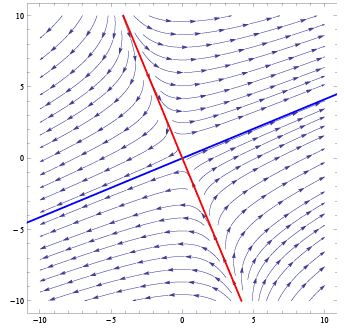Fig. 8.9. Phase portrait for dynamical system (8.20). Blue line represents unstable manifold, red line represents stable manifold.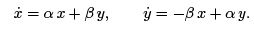(8.21)

Matrix of this system is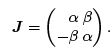(8.22)

System (8.21) is little trickier to solve. Let us switch to polar coordinate system by usual transformation

x = r cos θ; y = r sin  θ;

where r = r(t) and θ = θ(t). Inverse transformation reads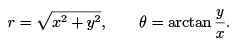These relations can be used to find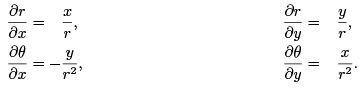Now we use (8.21) to derive corresponding equations for r and θ: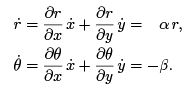We can see that dynamical system (8.21) in polar coordinates decouples to two independent equations for coordinates r and θ,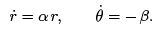(8.23)

First we solve equation for r. Let us write it in the form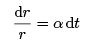which integrates to

log r = α t + log C

where the integration constant has been written as a logarithm (see footnote on page 162). Exponentiating the last equation we arrive at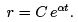Obviously, at time t = 0 we have r(0) = C and so we write the solution in the form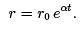Next we solve equation for θ. This is trivial since we have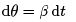which integrates to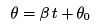where the integration constant has been denoted by θ0 and represents the value of θ at t = 0. Summa summarum, solution of system (8.23) acquires the form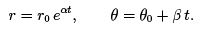(8.24)

Hence, solution of original system (8.21) in the Cartesian coordinates reads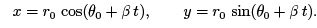(8.25)

Suppose that α = 0 so that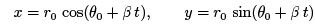Clearly, this represents motion at constant angular velocity β and constant radius r0 and therefore the phase trajectories are circles of radius r0. If
α ≠ 0, the radius of the "circle" will be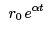and hence the trajectory will be a spiral. If α > 0, the radius will increase exponentially and the spiral will tend to in nity. If, on the other hand, α < 0, the radius will decrease exponentially and the phase tra jectories will spiral towards the origin. All cases are plotted in gure 8.10 by Mathematica commands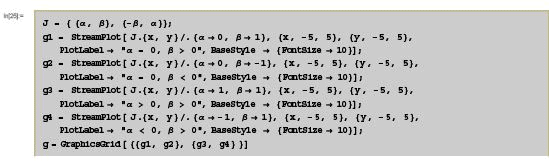and can be classi ed as follows:

• α = 0 Critical point is called centre. Tra jectories are circles centred at the origin.
• α > 0 Critical point is called unstable focus, tra jectories are spirals escaping to in nity.
• α < 0 Critical point is called stable focus, tra jectories are spirals tending to the origin.

Parameter β  has the meaning of angular velocity. If it is zero, spirals become straight lines and dynamical system reduces to previous case (8.17). If it is non-zero, its sign determines the sense of rotation: tra jectories orbit the origin in a clockwise sense for β  > 0 and in a counter-clockwise sense for β < 0.

Let us now analyse critical points of system (8.21) in terms of eigenvalues of matrix (8.22)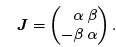We can use Mathematica to nd the eigenvalues and eigenvectors of matrix (8.22) by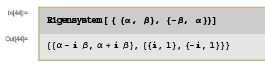which shows that this matrix has two eigenvalueswith eigenvectors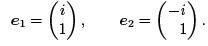In other words, eigenvalues and eigenvectors of matrix J satisfy relations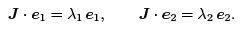The rst observation is that the eigenvectors are complex and hence there are no neither stable nor unstable manifolds, i.e. there is no real direction which is mapped to the same direction. The only exception is when β = 0 since in this case dynamical system (8.21) reduces to (8.17) and the eigenvectors become real.

Second, eigenvalues λ1,2 are mutually complex conjugated (as well as the eigenvectors),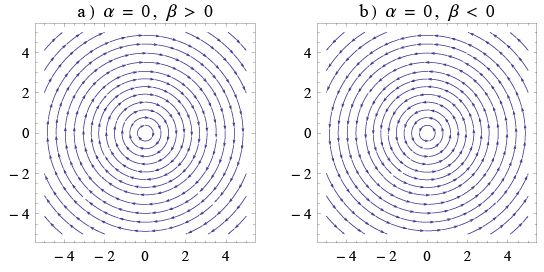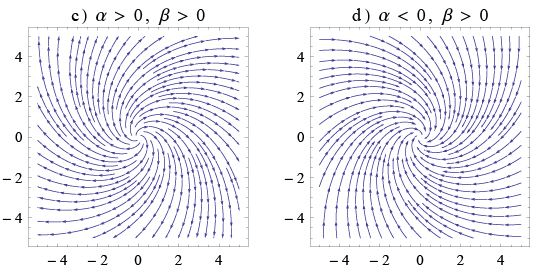Fig. 8.10. Classi cation of critical points for the system (8.21): a, b) centre, c) unstable focus, d) stable focus.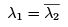where the bar denotes the complex conjugation. Hence, even if the dynamical system is not of the form (8.21), we can conclude, that if the matrix J has two complex conjugated eigenvalues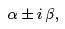the critical point is stable/unstable focus or a centre, depending on the values of α and β as classi ed above.

Example. Consider dynamical system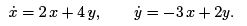This system is not of the form (8.21) but we can apply the criterion based on the analysis of eigenvalues. In Mathematica we type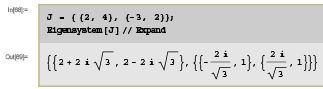where we have used Expand in order to simplify the expression for eigenvectors (try this code without Expand). We have found two eigenvalues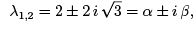which are mutually complex conjugated. In this case, parameters α and β are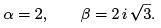Parameter α is positive and so the critical point is an unstable focus. Tra jectories of dynamical system considered: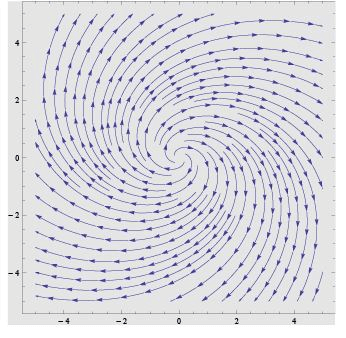Another example is the system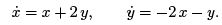Eigenvalues are found by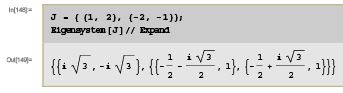Hence, now the eigenvalues are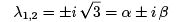which means that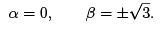Since α = 0, critical point is a centre rather than focus. Tra jectories of this dynamical system are the following: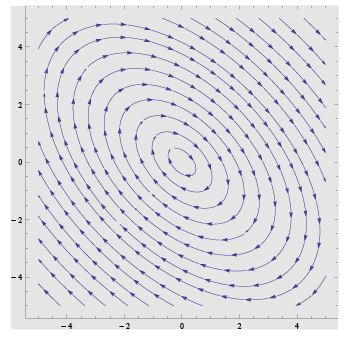General case

In the previous two sections we studied two special cases of planar linear dynamical systems given by matrices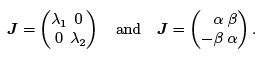However, we have seen that the analysis can be performed using the eigenvalues of these matrices. Now we consider general linear planar dynamical system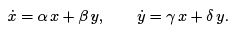(8.26)

Let us find the eigenvalues and eigenvectors of this general matrix. Recall that the determinant of matrix J is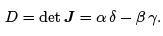The trace of the matrix is de ned as a sum of its diagonal elements, i.e.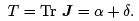Eigenvalues λ are defined by equation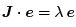where e is an eigenvector. The last equation can be rewritten in the form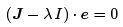where I is the unit matrix 2 x 2 so that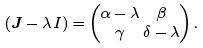This equation is a homogeneous system of linear equations which has non-trivial solutions only if the determinant of the system is zero: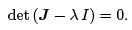Expanding the brackets we arrive at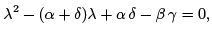or, equivalently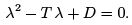This is a quadratic equation for λ and its solutions are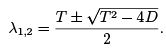(8.27)

Now we can summarize the classification of critical points as follows.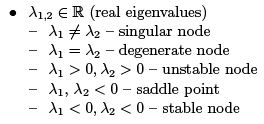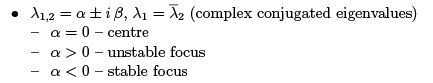Moreover, if the real parts of eigenvalues λ1,2 are non-zero, critical point is called hyperbolic, otherwise it is called non-hyperbolic.

Offer running on EduRev: Apply code STAYHOME200 to get INR 200 off on our premium plan EduRev Infinity!

159 docs

,

,

,

,

,

,

,

,

,

,

,

,

,

,

,

,

,

,

,

,

,

,

,

,

;